# Professional Development

## China England Exchange 2018-2019

London SW Maths Hub Primary Mastery Specialists, Ciara Sutton - Fitzpatrick and Ben Rankine  visited Shanghai in November 2018.  They shared their experiences in a daily blog which you can see here.

#### Showcase events

The London South West Maths hub once again was delighted to offer the opportunity to experience and observe first-hand Shanghai teaching practice in an English KS1 and KS2 classroom.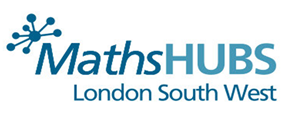## Primary Mastery Specialist Teachers Ciara Sutton-Fitzpatrick and Ben Rankine in Shanghai on the outward leg of the 2018-2019 England China Exchange.

### ENGLAND CHINA EXCHANGE 2018-2019 TEACHER BLOG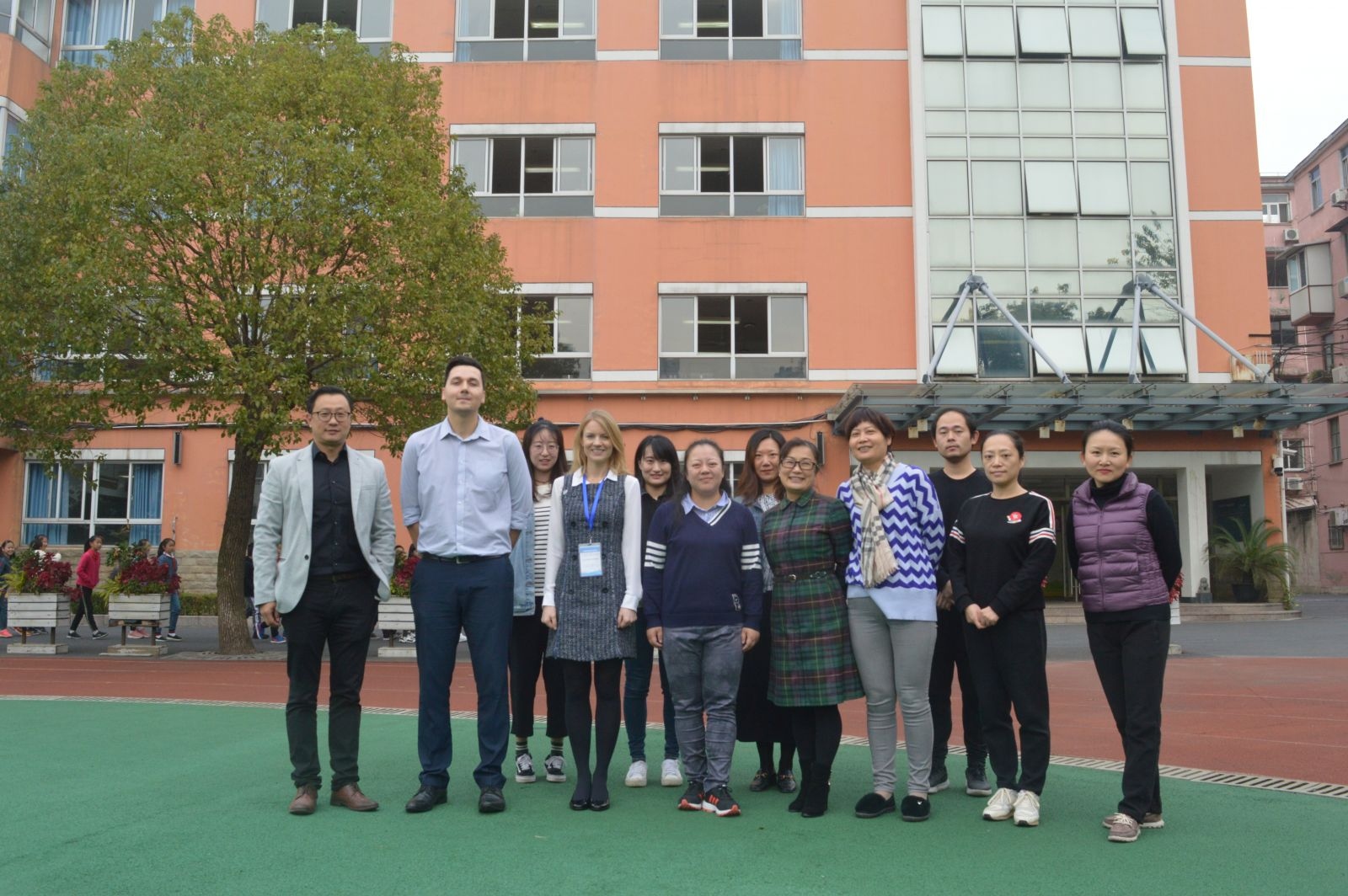## Day 8

Today we began our last day of observations in Shanghai. We were very excited for today’s lesson as we were observing a teacher who had many years of experience and in fact had taught our partner teacher earlier in her career.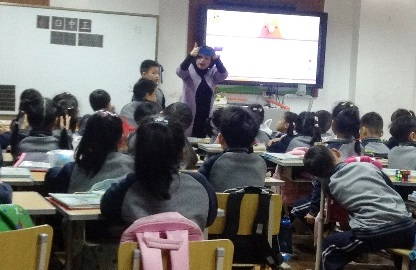Today’s lesson was a Grade 1 (Year 2) lesson on 3D shapes. The children had all brought bags of objects in from home as homework, which matched to the 3D shapes they were learning about (cubes, cuboids, spheres and cylinders). At the beginning of the lesson, the children were asked to name the different shapes on the board. This was the only prior knowledge they had of these shapes. They were able to name these shapes but had no understanding of what the characteristics of these shapes were.

The children worked in pairs to choose an object from the bag of objects they had brought from home. They would name these shapes to their partner to show they had the basic understanding of the 3D shapes.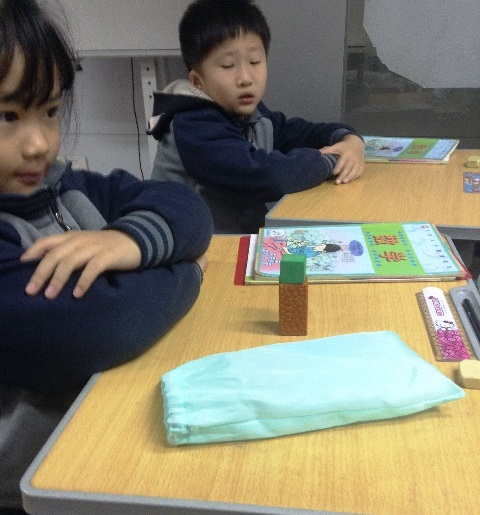They worked their way through each 3D shape looking at the characteristics. They explored how many faces the various shapes had, they looked at how many vertices the different shapes had. They also explored how the cylinder and sphere had round faces and how this was different to the faces that the cubes and cuboids had. There was really meaningful comparison between a cube and cuboid at this stage of the lesson. They realised these shapes were very similar, but made sure to explore the differences between the 2 shapes.At one stage, the teacher found a box which was very close to a cube in appearance but was in fact a cuboid. The discussion around this shape and why it was a cuboid and not a cube allowed the children to explore in real depth the characteristics of each shape

The exploration of misconceptions in this lesson was paramount. They looked at many cuboids which had similarities to cubes. One had 2 square faces and 4 rectangular faces, so they discussed why this would not be a cube. The teacher also found a cone. A cone was not a shape the children were learning, but they made sure to explore why this was not a cylinder to ensure their understanding of the cylinder was solid.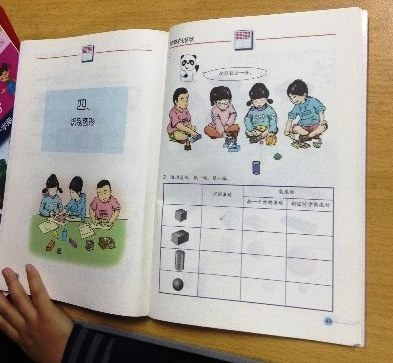The children had 2 tasks to complete the lesson. Firstly, they had to predict which of the shapes would roll and whether they would roll in one direction or many directions. The children had to make predictions before they were allowed to test this using the 3D shapes. They were able to summarise that the shapes with round faces were able to roll.

For the final activity of the lesson, the teacher presented 4 shapes on the board. 3 would be the same shape and one would be an odd one out (For example, there were 3 cuboids and 1 cylinder on the board). The children were expected to identify which shape was the odd one out and explain why it did not fit with the other shapes on the board.We really have enjoyed our time in Pingnan school and Shanghai as a whole. The experiences we have had here have been invaluable and we are now both very keen to return home and share all these wonderful ideas with our schools, our hub and our TRG schools.

## Day 7

This morning we watched a grade 5 lesson which focused on averages. This was an interesting lesson to watch because we had been told in discussions with the maths teachers that one of the aims in Shanghai is to provide children with more problem solving opportunities and also lots of real life context.

The teacher began the lesson with the following question:

I bought a box of oranges yesterday, but I can’t remember how heavy the box was.

He also had a large cardboard box full of oranges to show the children – we were impressed at this effort to make the problem really real-life.The teacher asked the children how the class could calculate the weight of the full box of oranges.

One pupil suggested that they could weigh one orange and then multiply the weight by the number of oranges in the box. Or that they could use a large scales to weigh the whole box. The teacher showed the children the scale – it was a small one and he said that this is the only one they had. The scales has a maximum capacity written on it so the children quickly realised that they couldn’t weigh the whole box of oranges.After this the children were put into groups and given some time to discuss a way to solve the problem. Afterwards, a child suggested that they could weigh 4 oranges and get the average weight of an orange and then multiply that to get the weight of the whole box.

Another child said you could weigh one orange and multiply it. The teacher asked were there any problems with this second suggestion. The class discussed that you would need to find a medium sized orange – an average looking one – for it to work.

A third method was suggested of weighing 5 oranges and finding the average value and then scaling up.The teacher gave the children some time to try out their various methods.

Then the class came back together and counted the oranges in the box which was 72.

The teacher then asked them to calculate the weight of the entire box of oranges.

The teacher brought the class back together for discussion. He asked for different group’s answers and recorded them on board.

The teacher put a table on the board to record the size of the samples (how many oranges) each group chose. The teacher then went to get a big scales to weigh the whole box.

The teacher recorded the exact weight of the whole box and wrote it on the board; this was 8.3kg. He then weighed the empty box which was 665g and subtracted that from the 8.3kg.

The class discussed whose answers were closest to the accurate answer and how this accuracy compared with the sample size.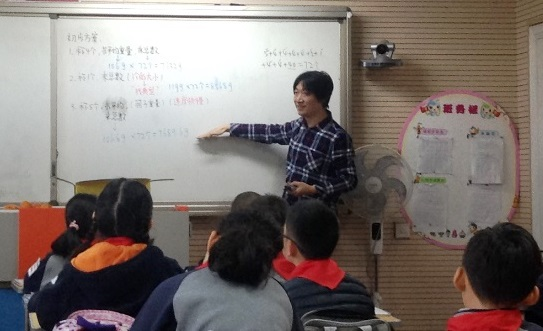There was an in-depth discussion about the different sample sizes and the effectiveness of different ones, for example whether an even number sample size was better to use than odd.

It was fantastic to see a lesson dedicated to solving one problem in depth. The children had already learned about averages and could apply their knowledge to a practical situation. We also enjoyed the focus on sample size and accuracy at the end of the lesson. The lesson reminded me of the analogy that we had heard at a maths hub training where you let the children off to explore and then take them back together. The children had the freedom to choose their sample size and method during the lesson but then the teacher carefully led the discussion at the end, focusing their attention on what he wanted them to notice.

## Day 6

Today we were lucky enough to see a grade 3 lesson at Pingnan school. Mrs. Zhou was teaching a lesson on the area of squares and rectangles.

She began her lesson by practicing times tables with the children. This was done orally by all children and they were practicing the same times table together. They were then presented with multiplication questions on the board. The questions required children to apply their understanding of times tables to solve the questions (e.g. I know 6 x 6 so I know 60 x 6). After a few minutes, one boy was asked to stand and read out his answers to the calculations. The rest of the children gave feedback on whether he had solved the calculations correctly or not.They then recapped their learning from the previous week. The children knew that they could find the area of a square or rectangle by doing length x width but did not have the understanding behind why this calculated the area of the shape. They had learnt that if they used squared paper they would be able to find the area of the shape. They had a fantastic tool for this, which was squared paper printed on plastic film. They were able to place this over any square or rectangle to find the area of the shape.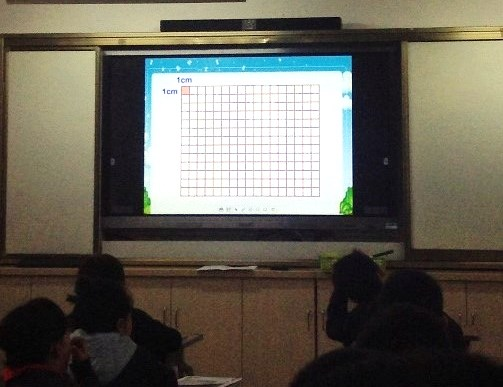The teacher pointed out that it took a long time to count each of the squares inside the shape (there were 54) and asked if they had any ideas about how they could find the area in a faster way. On child mentioned that there were 6 rows of 9 and another talked about 9 columns of 6. They were making the link that they could use multiplication to find the area of the shape and it was a lot faster than counting each of the squares individually. At this stage, the children knew that there were 54 squares but were not aware of the unit of measurement for area. The teacher discussed how area was measured in square centimetres and therefore the area of the shape was 54cm2.The children were then presented with 2 different rectangles. The teacher asked the children to compare the area of these 2 shapes. The expectation was that the children would place the grid over the shapes and count the squares. They realised that although these shapes looked different, they in fact had the same area.

They proceeded to look at 2 rectangles without the use of squares. The teacher was looking for the children to apply their understanding of the formula to find the area of a shape that was 9cm by 6cm and another that was 5cm and 4cm. The children used their secure knowledge of times tables to find the area very quickly, but the teacher’s questioning took them deeper with their learning. She aske what the 9 meant, looking for the children to realise there were 9 squares in a row. This questioning continued with what the 6 meant and the children stating that there were 6 rows of 9.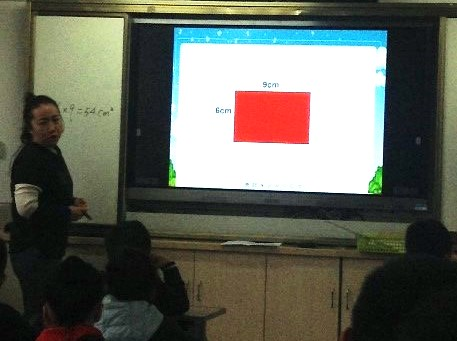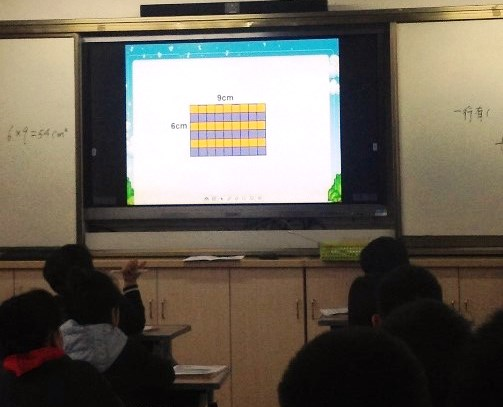The teacher moved the lesson entirely into the abstract. She asked if a shape was 10cm by 6cm what the area would be and what the shape would look like. The children visualised this shape in their mind. They even drew the shape in the air with their fingers to show they were imagining it! She then asked what if it was 8cm by 6cm. Again the area was calculated easily, but she asked the children to explain why the area was now smaller than the previous shape. This questioning made the children reflect deeply on the relationship between length, width and area.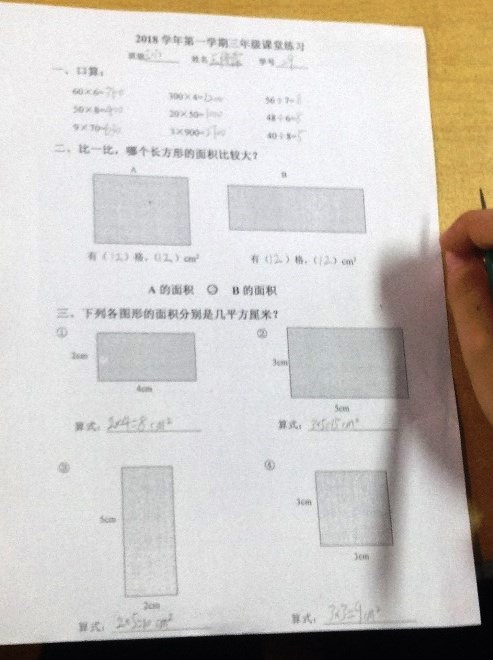It was now the opportunity for the children to practice their learning from the lesson by calculating the area of 4 rectangles on a sheet. They were required to write the full calculation out to show they fully understood how to apply the formula to find the area of the shape.

The lesson ended with a reflection and an exploration task (Dong Nao Ting). The children re-stated what they had learnt today and the key points of the lesson. Their exploration task was to draw a rectangle that had an area of 12cm2. The teacher made it clear to the children there would be more than one possible solution to this challenge and they would need to explore this problem. They found many solutions, including one that was drawn diagonally across the squares. They had a look at this answer and went back to the counting squares method to prove that this child had in fact found a correct solution.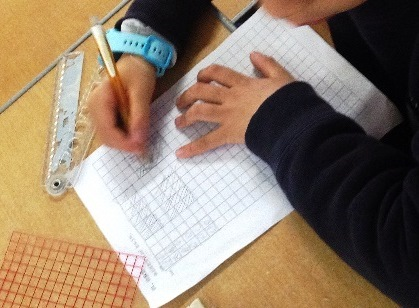It was very interesting to see the Chinese take on a concept that many children struggle with in British education and we found many ideas to trial in our own classrooms.

## Day 5

Today we arrived at Pingnan Primary School where we were warmly welcomed by our host, Louise, and her colleagues. We begun our day with a grade 1 lesson which focused on addition to 20. We were told that the children had only calculated within 10 before and this would be their first day adding numbers which totalled more than 10. This lesson was also a prizewinning lesson so we were really excited to see the learning journey!

When we got to the hall, tables were already set up with resources which we later learned had been designed by the teacher! The teacher asked the children to put 9 blue beads to the centre and 4 red beads to the centre with a little gap between the red and blue beads. This resource was then left to the side until needed later in the lesson.The lesson began with a warm up game – teacher said a number and children had to show that number on their fingers. They represented the numbers using the “5 and a bit” relationship which was key to the next 2 activities.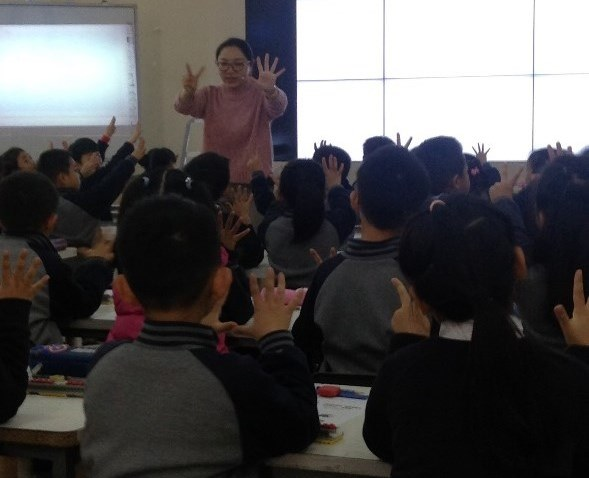The teacher showed a picture of counters on the board and children had to say what the number was. The picture only flashed up for a second or two but the numbers were represented in a way that children could quickly identify the number.

The first picture is 7 which is represented as a row of 5 then a row of 2.

The second picture is 13 represented as 2 rows of 5 and a row of three.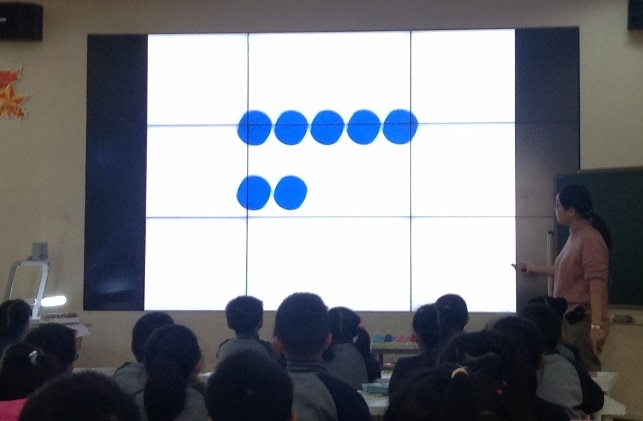The teacher then showed the equation 5 + 5 = 10 as an equation to help children with calculation. She asks them where they can find this equation on their body e.g. hands.

The teacher then explained that today’s topic is addition to 20 and showed the following calculations:

6 + 6 =

7 + 7 =

8 + 8 =

9 + 9 =

One by one the children solved each equation using their hands. For 6 + 6 they make 6 with 5 fingers on one hand and one on the other. They are then told to double both hands so that the calculation becomes double 5 + double 1.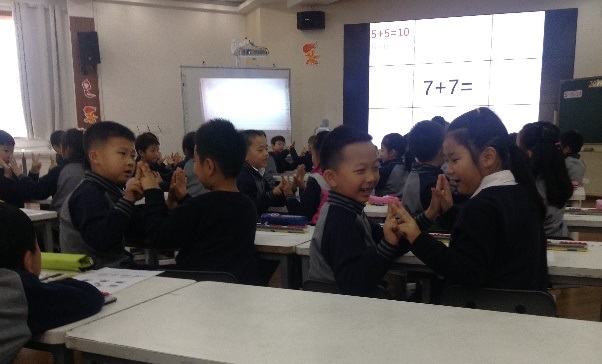The teacher asked different children to explain each equation and they were encouraged to use the stem sentence: 5 + 5 = 10 and 2 + 2 = 4 (or relevant number) so the total is 14.

The teacher also took pairs up to the front to demonstrate this method together. The pair stood facing each other with their hands against their partner so that the number of fingers on each hand was doubled. This was a lovely concrete way of showing the calculation! The children continued to use the stem sentence as above.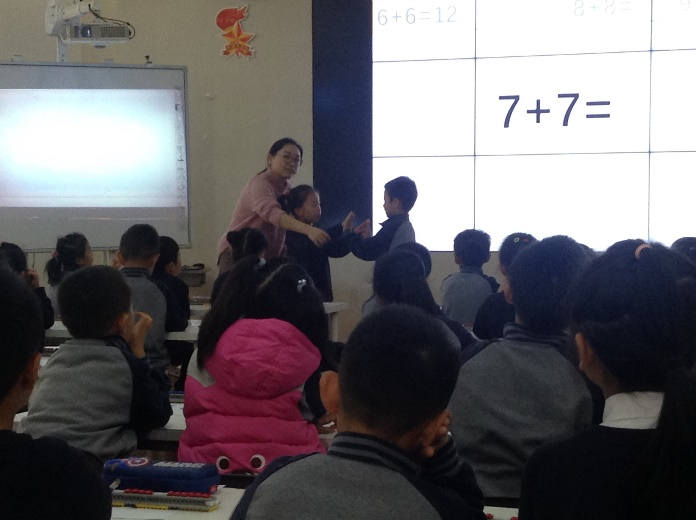The teacher explained that this is one way to do these calculations. She now asked them to imagine the number without using their fingers and asked them to visualise the calculation. They discussed how one of these calculations could be done mentally. It was so clear to see the move from concrete methods to abstract learning.Then the teacher showed the calculation 9 + 9 = and asked pupils for another way to solve this. A pupil suggested a method which we would call bridging through 10 or a “make 10 strategy” : 8 + 2 = 10 and another 8 is 18.

The teacher showed a representation of this on board – see photo. The representation uses colour to show how a red bead joins the blue beads to make 10 and then the other red beads are added to make 18.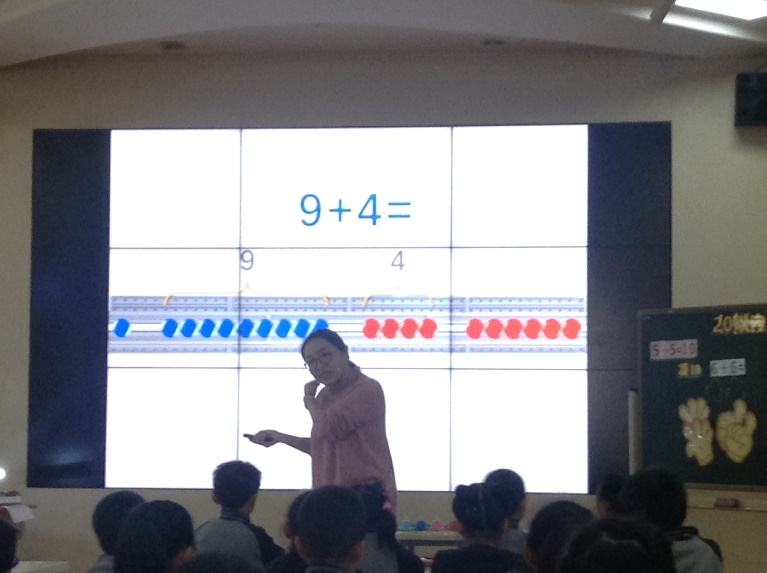The teacher then discussed with the class the difference between the two methods. The doubling method works when the two numbers are the same. The bridging method works well when one number is 9 and a different number is being added. The students were clearly being encouraged to consider why each method was more effective than another in a certain situation.

The teacher asked children to do 9 + 4 and brought a child up to the board to explain how to work it out using this “make ten” strategy.

The children then worked out the same question using another method – add one blue bead and take away one red bead. Therefore the calculation became 10 + 3. Children discussed this with their partner.

More practise was then carried out on the bridging through ten method.

A child came up to explain how to work out 9 + 7. He uses the bridging method where he adds one red bead to the 9 blue beads and then adds another 6 red on.

The teacher then puts the equation on the board 9 + 7 = 16 and asks the children if we know this can we also work out 9 + 8 and 9 + 6 . This was a fantastic example of procedural variation and how children are being encouraged to use knowledge they have to work out other calculations.

The first child to offer an answer explained how to do 9 + 6 using the bridging through ten method.

The teacher asked for another method and a girl stood up and explained that if we know 9 + 7 = 16 , 8 is one more than 7 so we can just add 1 on.

Then the teacher showed the following calculations on the board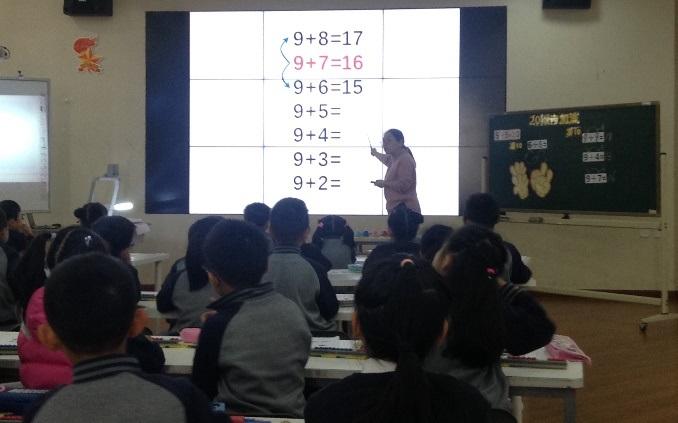Now teacher asked them to do 9 + 5 mentally and they can choose the method. A boy explained that you can separate 5 into 1 and 4 and then add the 1 to the 9.

The teacher showed the following picture and discussed the steps which need to be taken.

• First step is to fill in the box
• Second step is to circle 10 (9 from one group and 1 from another)
• Third step is to add 10 and 1
• Fourth step is to explain what they’ve done.After this they used the same method to practise the following calculations.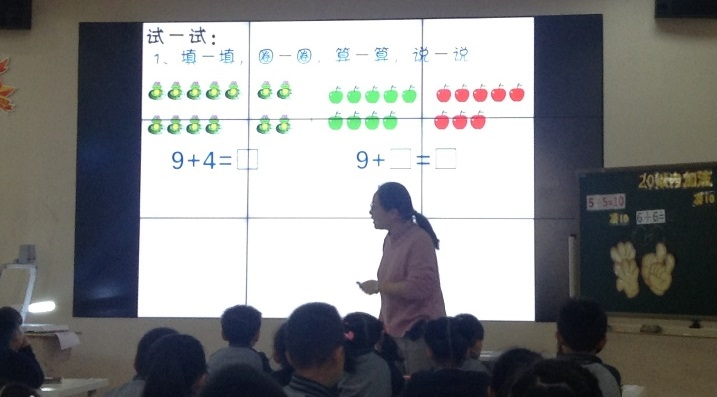The teacher showed 2 children’s work who have both been correct but done it slightly differently. She discusses with the class the methods and why they work.

The children tried the joining up exercise on their sheets – see photo below. Once they had finished there was a class discussion about it, in particular the 8 + 7 calculation (as this was not one of the main teaching points today).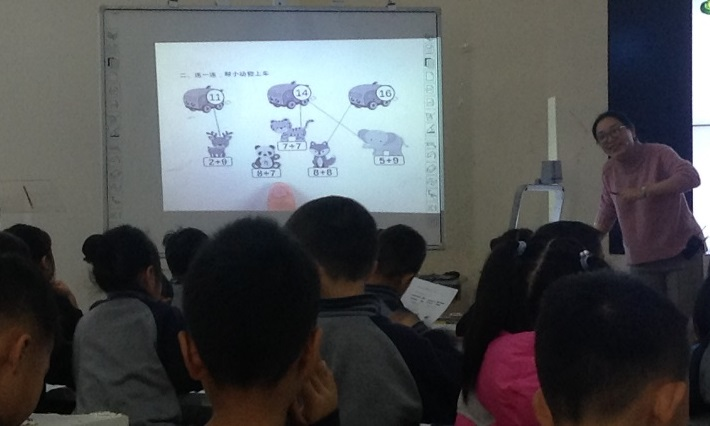They identified three ways to solve this calculation.

8 + 7 + 1   (near doubles)

8 + 8  - 1 (near doubles)

8 + 2 + 5  (bridging)

Straight after the lesson we had a TRG style discussion with a group of maths teachers, including the teacher who had taught the lesson. I was curious as to the prior learning and subsequent learning of this class. We were told that this was their first lesson calculating over ten and as seen in the lesson, the focus was doubles and adding to 9. The next day the focus would be adding to 7 and 8 – a preview was seen in the extension question at the end of the lesson.

We are very much looking forward to more maths lessons tomorrow!

## Day 4

Today we concluded our journey with Changqing School. Mr Lu’s maths class used today’s session to mark and correct their home work from the night before. This was an interesting exercise to see as under the time pressures of an English school, there may not always be the opportunity to go through the homework as thoroughly as we observed today. It was clear that the practise work the children did at home was a valuable part of their learning journey. The tasks, which were in the practise book that is used alongside the textbook, were very clearly aligned to the lessons we had seen and included a mix of question styles.Mr. Lu began this session by reviewing the learning so far about parallelograms. He drew a parallelogram on the board and proceeded to work through a number of the key points related to the shape that they had learnt so far. These included:

• Labelling the shape ABCD and discussing how it could be named
• How opposite angles in the parallelogram are equal
• How the opposite edges of the shape are equal in length
• How the opposite edges of the shape are also parallel
• That the interior angles would add up to 360o

What was most interesting about this segment of the lesson was that Mr. Lu made numerous mistakes deliberately whilst discussing the prior knowledge. The children were quick to pick these up and correct these really showing their understanding and depth of knowledge.The rest of the session was used to mark the homework with the children. Almost all of the tasks were built around the same activities the children had completed in class that week, meaning what they had done at home shouldn’t have been challenging but rather provided the opportunity for the children to consolidate their learning. The tasks included in the home learning were:

• Labelling which edges of the shape were parallel
• Naming the parallelogram ABCD
• Diagonal line through the shape
• Understanding the two triangles inside the parallelogram are equal
• Ticking the characteristics of squares, parallelograms and rectangles
• Understanding a square is a special rectangle and a rectangle is a special parallelogram
• Stable triangles and unstable parallelograms
• Ticking which shape is a parallelogram
• Complete the shape so it is a parallelogram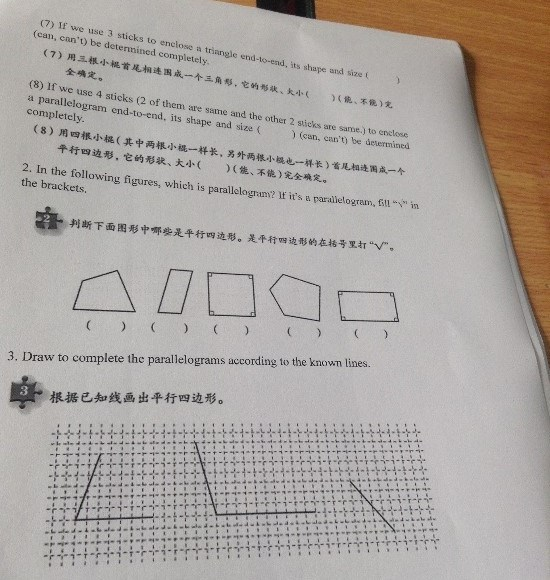The final part of the home learning we identified as the Dong Nao Ting (or extending exercise). The children were presented with a trapezoid filled with equilateral triangles. They then had to see how many parallelograms they could find inside the shape. This was a rich task that really gave the children the opportunity to explore the parallelogram as a concept. Mr. Lu discussed the strategies for finding all of the parallelograms in a systematic way.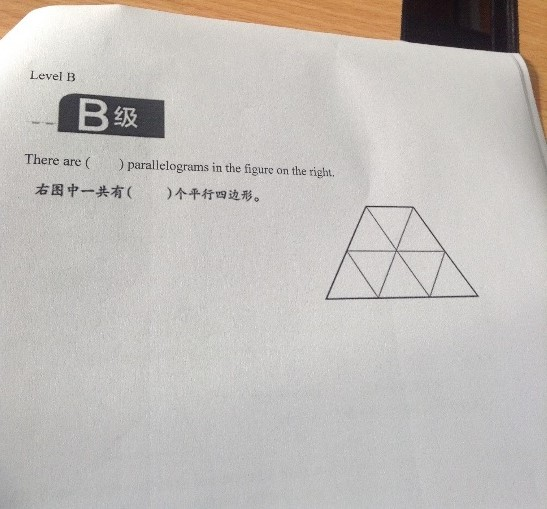The real value in this marking and correction exercise came through the discussion of mistakes. Mr. Lu would ask after each question if anyone was wrong and if they were, they would raise their hand. There was no stigma or shame around this; they valued the learning experience. They would discuss the mistakes made and unpick why this had happened.

We really valued our time at Changqing School. We learnt a lot during our observations and have come away with a wealth of ideas around maths. The school were very accommodating and gave us ample opportunities to integrate with the Chinese culture as well. Next week, we will be visiting another school and look forward to the experience that will bring.

## Day 3

Today we continued our journey with Mr. Lu through parallelograms. We have been amazed by the time and dedication given to fully understanding this 2D shape so far.

The children began by recapping their learning so far. They discussed what a parallelogram was, the characteristics of the shape and the relationship between the parallelogram, square and rectangle. We could really see how the depth of learning on previous days had enabled the children to so confidently discuss their learning with one another.

The children could explain the following three characteristics of the parallelogram:

1. The parallelogram has 2 opposite sides.

(Teacher tells all children to touch the opposite sides of their parallelograms)

1. The 2 opposite sides are parallel.
1. The length of the opposite sides are equal.

The children then made use of concrete resources to continue their learning. They had 2 parallelograms they had cut out from previous days and proceeded to label the vertices as ABCD.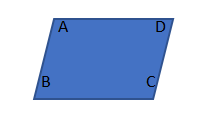After drawing a line through the centre of each parallelogram to connect different vertices, they cut the parallelograms into triangles. They were then asked to explore these shapes and explain what they had noticed. The attention was drawn to the fact that when you cut A->C you get 2 equal triangles. If you cut B->D you get 2 equal triangles. However, the triangles made from AC and BD were not equal with each other. Using this understanding the children were able to understand that the opposite angles of the parallelogram must be equal.

This was added to the list of characteristics on the board

4. The opposite angles of a parallelogram are equal.

The lesson continued with the use of concrete manipulatives as then children selected 4 sticks from their tables to make a parallelogram. The sticks were of varying length and there was discussion about which sticks to choose and why. The children quickly recalled the fact that the parallelogram must have 2 pairs of equal sides and therefore they would need to ensure they had sticks of matching length. As the children built parallelograms from the sticks, Mr. Lu photographed their work and it appeared on the white board. Both of use were amazed by this technology and the applications it had for prompting classroom discussion.

The discussion now centred around the fact that the parallelograms all looked different, and could they all be correct? They generalise that the parallelogram is unstable, meaning that the angles and the area of the shape change whilst the length of the edges remain constant. They looked at a real life example involving a closing gate (where the railing was in parallelogram shape which changed as the gate moved) and also compared this to a triangle (which they also built from sticks) where they could not change the angles and area without changing the length of the sides.

The children concluded the lesson by completing some exercises. Initially, they had to find the missing interior angles of the parallelogram and use this to calculate the total interior angles.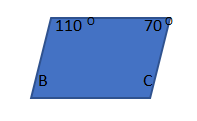They applied their knowledge from the lesson to find angle C and angle B and explained how they worked them out. Then they were asked to add up all the interior angles and came to the conclusion that the interior angles would add to 360O. In the next problem, they knew only one angle.

They quickly realised they could work out the opposite angle and then used many different methods to find the remaining angles. These included:

• Adding the two known angles and subtracting this from 360o. Then halving this to find the 2 missing angles
• Knowing 2 of the angles would make 1800 so subtracting the known angle from 180o
• Splitting the shape into 2 triangles and using this to find the missing angle.

We were impressed by the confidence in the range of methods and realised how much time must have been spent teaching triangles and angles in previous years. We look forward to seeing the next part of Mr. Lu’s unit on parallelograms tomorrow when we conclude our visit to Changqing School.

Note: We would like to have shown photos of some of the examples and slides; unfortunately, due to technological issues we can only describe them. Hopefully our photos will be back tomorrow!

## Day 2

Today we were lucky enough to see the second lesson on parallelograms by Mr. Lu. In the previous session the children had been introduced to the concept of parallelograms and begun to explore the characteristics of the shape. They began today’s session by looking back at the various types of quadrilaterals they knew (squares, rectangles, parallelograms).Mr. Lu explained that today the children would be exploring the characteristics of these shapes. They looked at how 2 overlapping rectangles could be used to form each of these shapes (and also a rhombus, referred to as a diamond by the children in the class). Mr. Lu made a point that this was a special shape they would learn about in Middle School. He didn’t shy away from this shape but also was not exploring it within this lesson.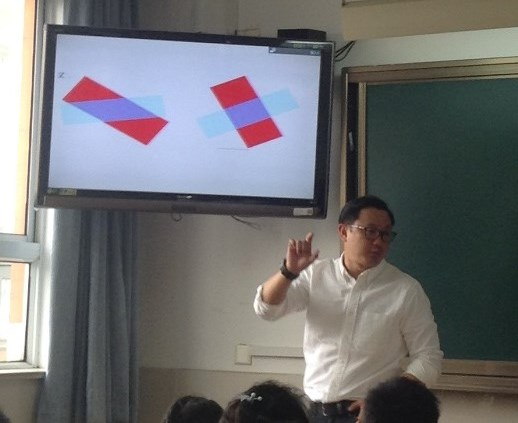The first task of the session involved the children measuring the edges of a parallelogram. They would then complete a stem sentence to show they had understood the key characteristics of the shape. This would then be fed back to the class as part of a discussion on what the characteristics that made the shape were. These key facts were repeated by many children, read out by the girls in Mandarin and the boys in English. This was a fantastic example of how repetition can be used to engage all children with the key points being taught.We then saw a child-led example of procedural variation. Mr. Lu asked his class how they knew the opposite edges of the parallelogram were equal. Three different children offered responses on how this was done ranging from measuring with a rule to drawing vertical lines inside the shape as proof. This particular child was recalling their knowledge from Grade 2 in applying this method.

They proceeded to measure the edges of a square and a rectangle and discuss the key characteristics of each shape in the same way they had the parallelogram. There were many opportunities for discussion between the children about what they had discovered at each stage of the lesson.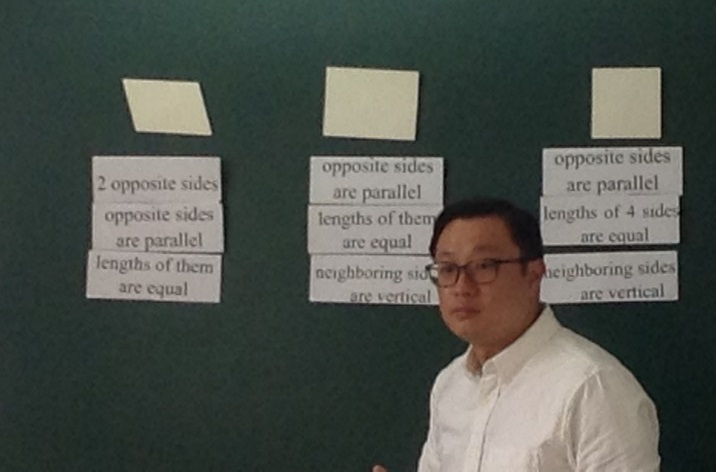After the children had discovered the characteristics of the shapes, they moved onto their second learning point of the lesson “What is the relationship between these shapes? They began by comparing the parallelogram and the rectangles. The children identified each of the similar properties such as opposite sides being parallel and opposite edges being equal lengths, but also were able to identify that the rectangle had vertical edges (referred to as neighbouring edges are vertical).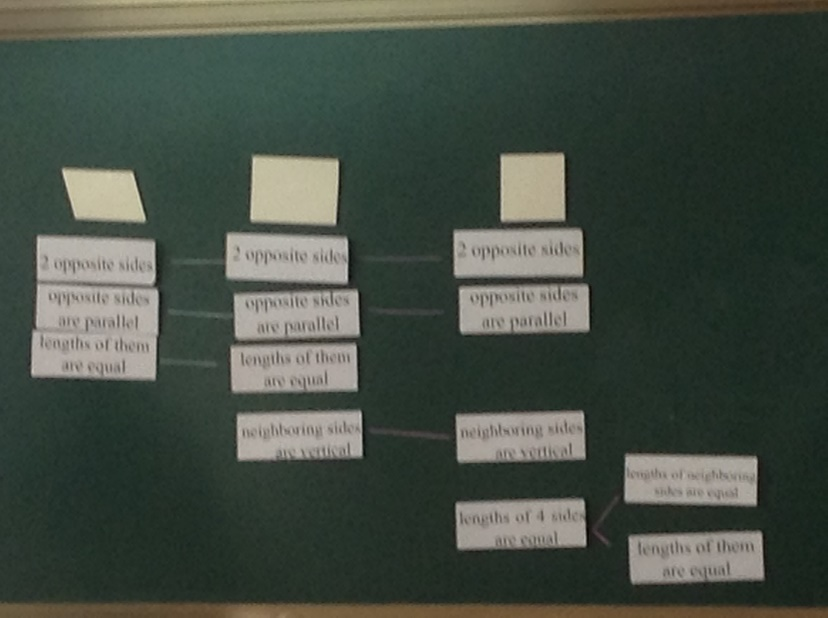They continued their comparison by looking at squares and rectangles before generalising the links between each of these quadrilaterals. A lot of emphasis was placed on comparing these shapes and drawing out the essential features of a parallelogram. It was lovely to see the children come to their own conclusions about what makes a shape qualify as a parallelogram.The session finished with what was close in meaning to a Venn Diagram. It showed how a square was a special rectangle and a rectangle was a special parallelogram.Overall this lesson was a great follow on from yesterday’s lesson and it was clear that time was being given to this shape for the children to really explore it in depth.

## Day 1

We started off our day in Changging Primary school with a very warm welcome from the headteacher, our partner teacher (Lu), and several other teachers. We were then presented with a beautiful bouquet of roses by 2 children who told us how delighted they were to have us in their school and to learn “the beauty of mathematics” with us. After a brief stop and cup of tea in the library, we embarked on a tour of the school. We were lucky enough to be invited to look in on a calligraphy lesson and an embroidery lesson – we even had a go at each! Soon it was time for the maths lesson which took place in Lu’s grade 5 class (equivalent to year 6 in England).

The lesson focused on parallelograms as part of the shape unit. The specific learning objective was to identify a parallelogram. The lesson began with a recap of prior knowledge and children were asked what shapes they had already learned about; they replied a circle, square, rectangle and triangle. They also traced these shapes with their hands to show their understanding of them.

Then they spoke about lines – straight line, parallel lines, ray, line segment, perpendicular lines.#### New learning

The teacher asked the children to draw a pair of parallel lines which they did in their exercise books. The teacher then showed a set of parallel lines on the board – both horizontal. He asked whose lines were like his. He then asked the children to draw another set of lines in a different direction.

After they did that , the teacher showed 2 sets of parallel lines overlapping and asked,” Can you find a new figure in the screen?” The children were instructed to colour in the shape in between the lines.  The teacher asked “What’s this shape?” and the class agreed it was a parallelogram. He told them that today they would learn to recognise this figure.The teacher asked, “Do you have any knowledge about this figure?” and one child replied that there were 2 pairs of parallel lines in it.

The children were given 5 minutes to read the next page of their textbooks and afterwards discuss what they learnt. D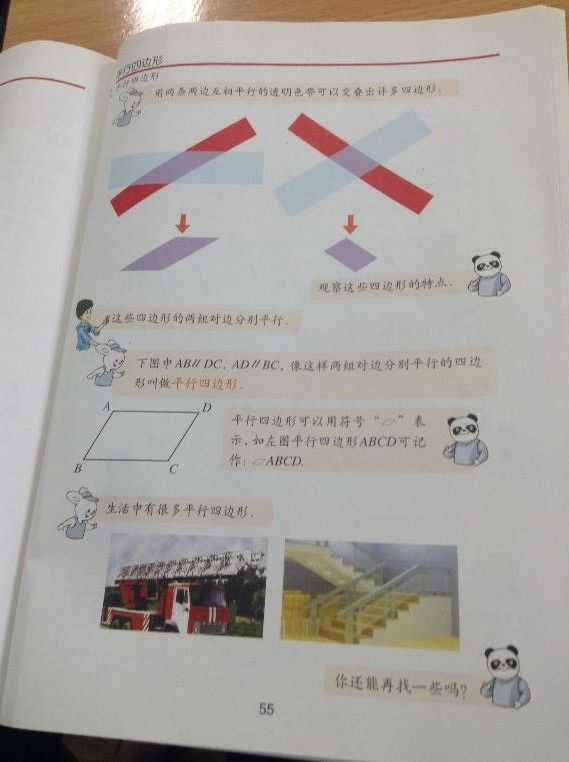The teacher then showed a parallelogram labelled ABCD. The class discussed how AB is parallel to DC and AD is parallel to BC.

The teacher said that we can call this parallelogram ABCD. What else can we call it?

The children gave suggestions eg ACDA. The teacher asked, “Can we write it like ACBD?”

There was a discussion about how you couldn’t call it this because AC is not a line they are opposite corners. (non-concept) C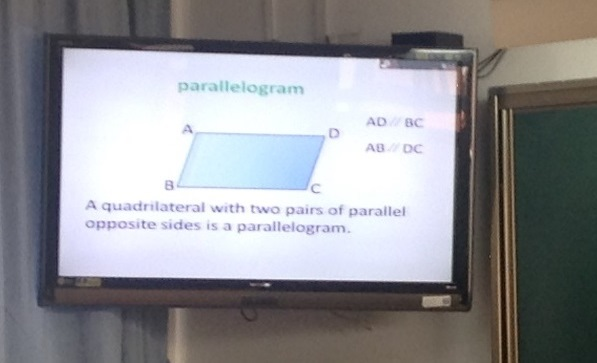Next we saw a great example of conceptual variation and the use of the non-concept. The teacher asked which of the following figures are parallelograms? C

The first was not because it only had one pair of parallel lines. The second one was not because although it had 2 sets of parallel lines, the shape had more than 4 sides. The third shape had 2 sets of parallel lines but one line did not meet the other so it had no corner. The fourth was a parallelogram. The children explained their reasoning for every shape.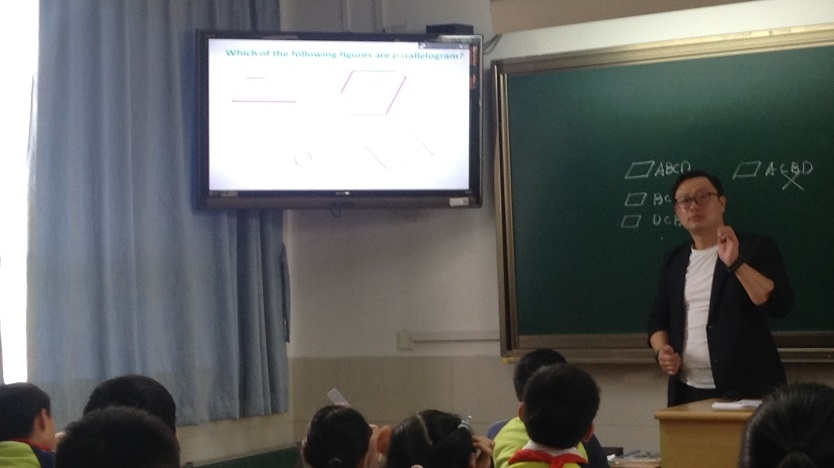Next the teacher asked where you could see a parallelogram in daily life and showed some pictures of the shape in their school.

Then children were asked to draw another parallelogram EFGH in their books. When they had done this, a child explained the necessary steps to draw a parallelogram. Attention was drawn to the fact that if one line is 8cm, its parallel line must also be 8cm. G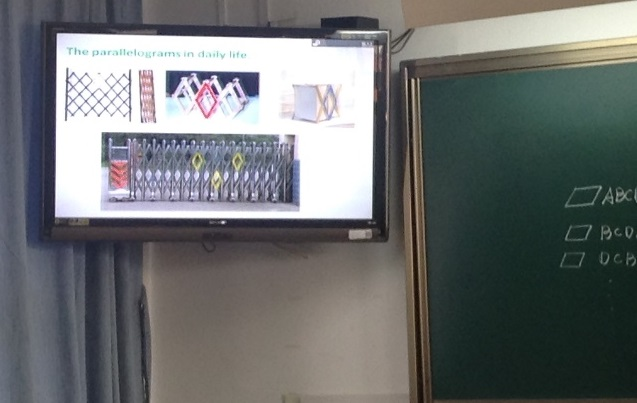Finally children draw another parallelogram and cut it out.

In this lesson it was easy to spot the conceptual variation, the pre-empting of misconceptions, the children moving at the same pace, the general class discussions, the ping pong style of teaching  and the precise use of vocabulary.

We are looking forward to seeing the next lesson in the unit tomorrow!